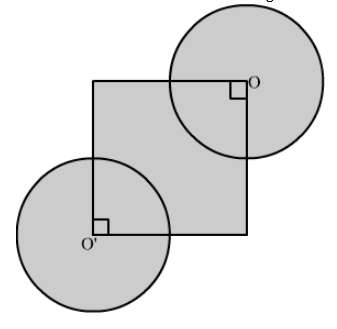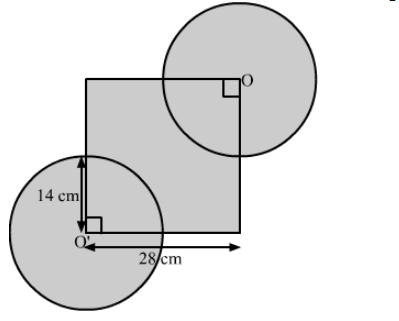# In the given figure, the side of square is 28 cm`
Question:

In the given figure, the side of square is 28 cm and radius of each circle is half of the length of the side of the square, where O and O' are centres of the circles. Find the area of shaded region.Solution:

We have,

Side of square $=28 \mathrm{~cm}$ and radius of each circle $=\frac{28}{2} \mathrm{~cm}$= Area of the square + Area of the two circles − Area of the two quadrants

$=(28)^{2}+2 \times \pi \times\left(\frac{28}{2}\right)^{2}-2 \times \frac{1}{4} \times \pi \times\left(\frac{28}{2}\right)^{2}$

$=(28)^{2}+\frac{3}{2} \times \pi \times\left(\frac{28}{2}\right)^{2}$

$=(28)^{2}\left(1+\frac{3}{2} \times \frac{22}{7} \times \frac{1}{2} \times \frac{1}{2}\right)$

$=(28)^{2}\left(1+\frac{33}{28}\right)$

$=(28)^{2} \times \frac{61}{28}$

$=28 \times 61$

$=1708 \mathrm{~cm}^{2}$

Therefore, the area of the shaded region is 1708 cm2.